# Estimating Policy Barriers to Trade

## Free and Fair?

“We believe that trade must be fair and reciprocal. The United States will not be taken advantage of any longer.”
$$\qquad$$ – Donald Trump, Feb. 2019

“I’m going to have a trade prosecutor…to make sure every trade deal we’re already in is absolutely followed to the letter so nobody takes advantage of us.”
$$\qquad$$ – Hillary Clinton, Oct. 2016

“Internationally, we are seeing a tendency toward protectionism and navel-gazing…What we need is trade that’s both fair and free.”
$$\qquad$$ – Shinzo Abe, Oct. 2016

“An open world is only worth it if the competition that takes place there is fair.”
$$\qquad$$ – Emmanuel Macron, Sep. 2017

• Free: Foreign firms enjoy same market access conditions as domestic competitors
• Fair: Barriers that do exist affect all trade partners symmetrically

## The Tariff System (GATT/WTO)

• Free? Applied tariff rates are low, ~5% on average (Baldwin 2016)
• Fair? WTO members (vast majority of world economy) commit to principle of non-discrimination (Most Favored Nation)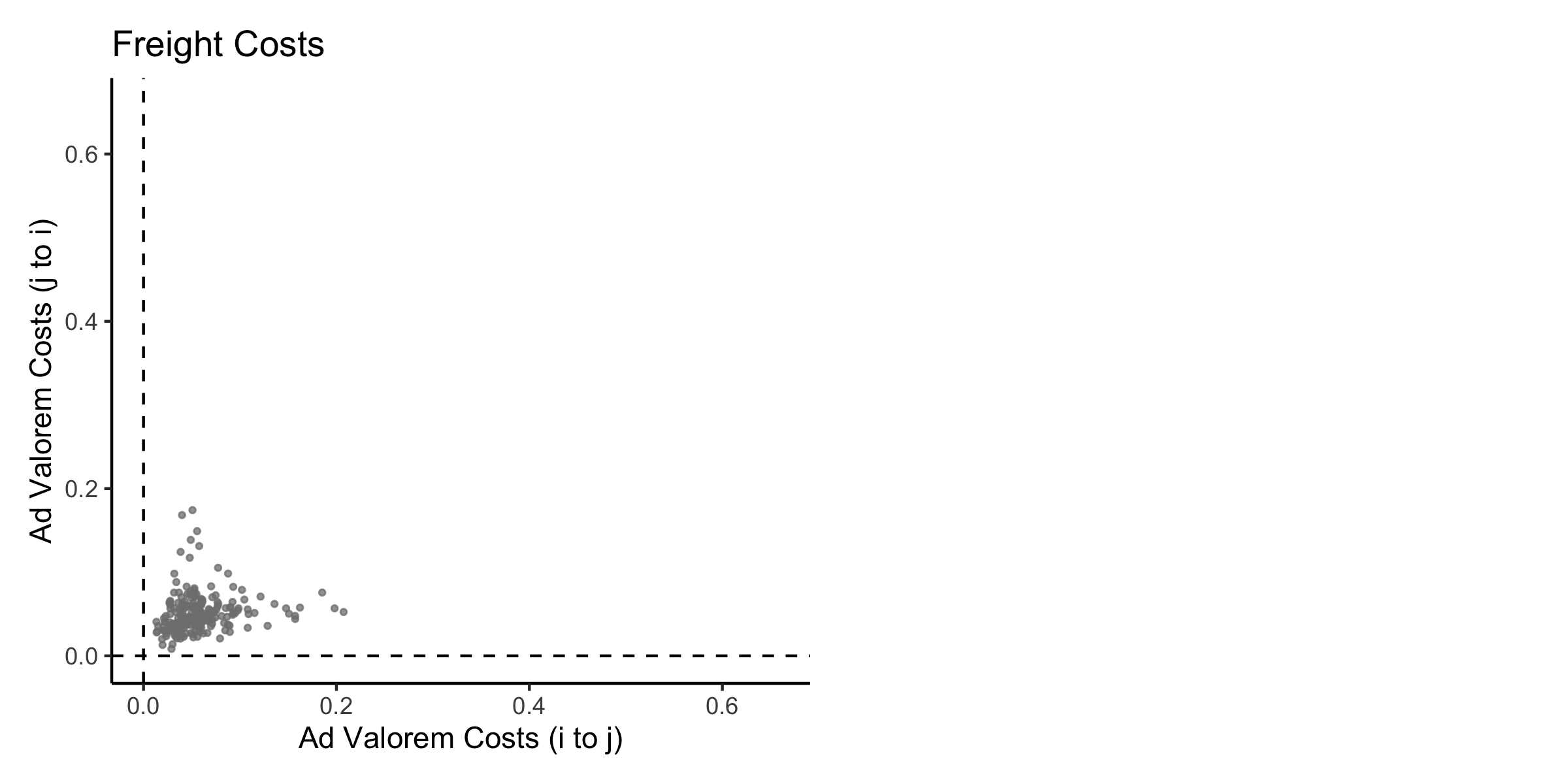## The Tariff System (GATT/WTO)

• Free? Applied tariff rates are low, ~5% on average (Baldwin 2016)
• Fair? WTO members (vast majority of world economy) commit to principle of non-discrimination (Most Favored Nation)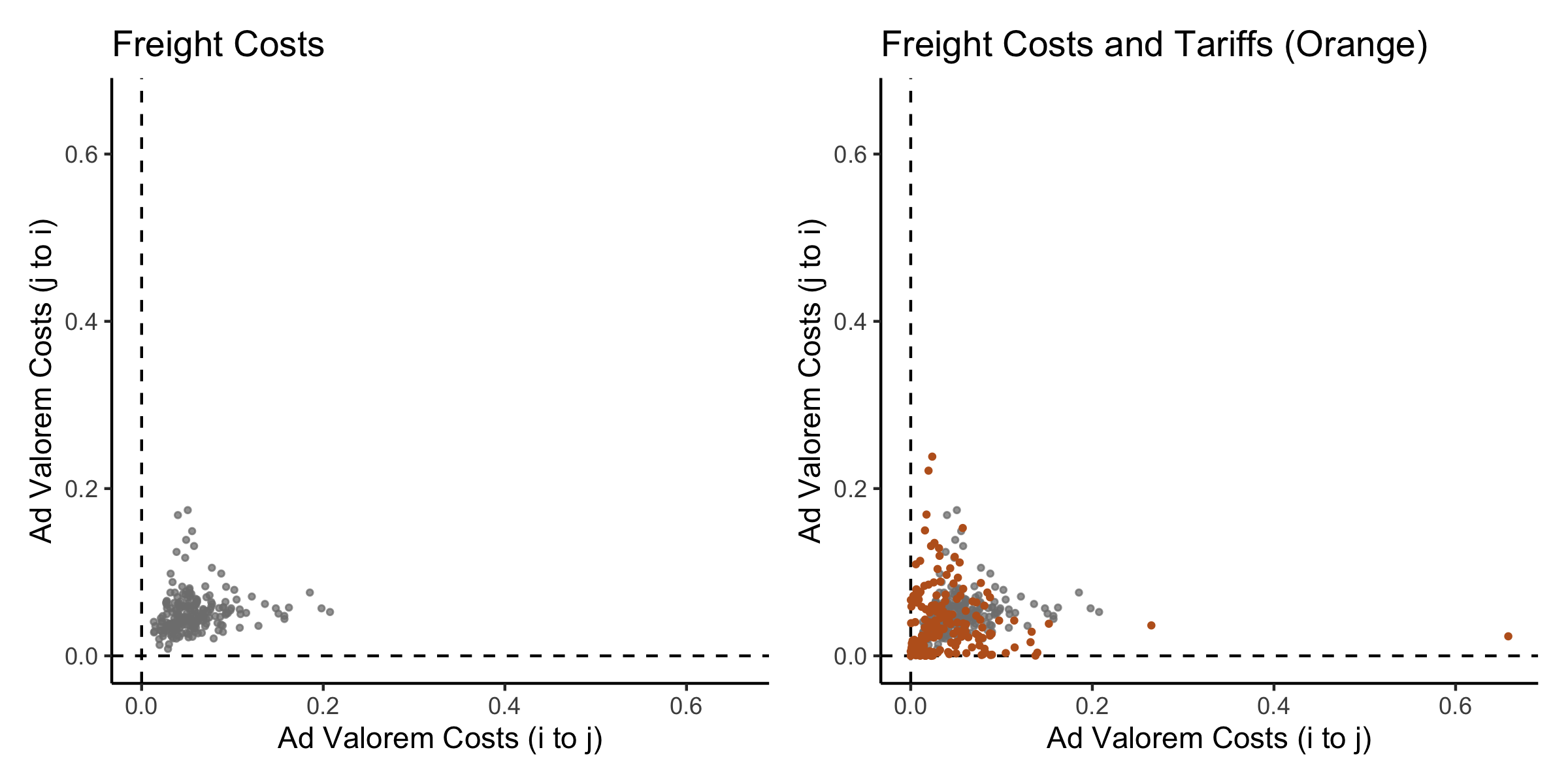## Varieties of Barriers

Direct

• Tariffs
• Non-Tariff Measures (NTMs)
• Price controls, quotas, health and safety regulations, technical barriers
• Mansfield and Busch (1995), Lee and Swagel (1997), Gawande and Hansen (1999), Kono (2006), Rickard (2012), Maggi, Mrázová, and Neary (2018)

Indirect (Behind-the-Border)

• Government procurement
• Evenett and Hoekman (2004), Kono and Rickard (2014)
• Subsidies
• Excise taxes
• Regulations

Effective Discrimination: Target trade-disorting instruments to disproportionately affect disfavored trading partners.

• E.g. high agricultural duties by developed countries disproportionately harm developing countries

## Measurement Matters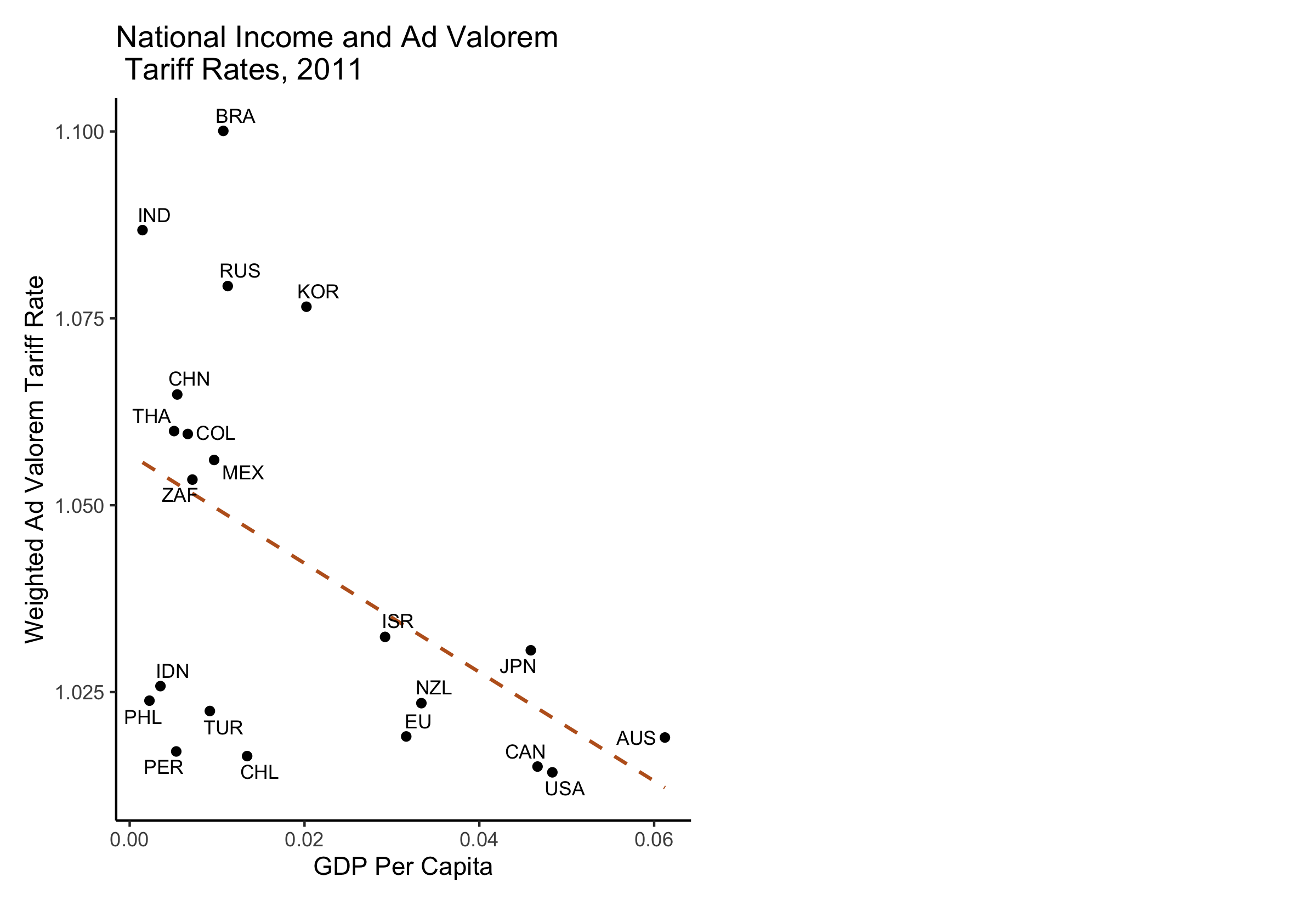## Measurement Matters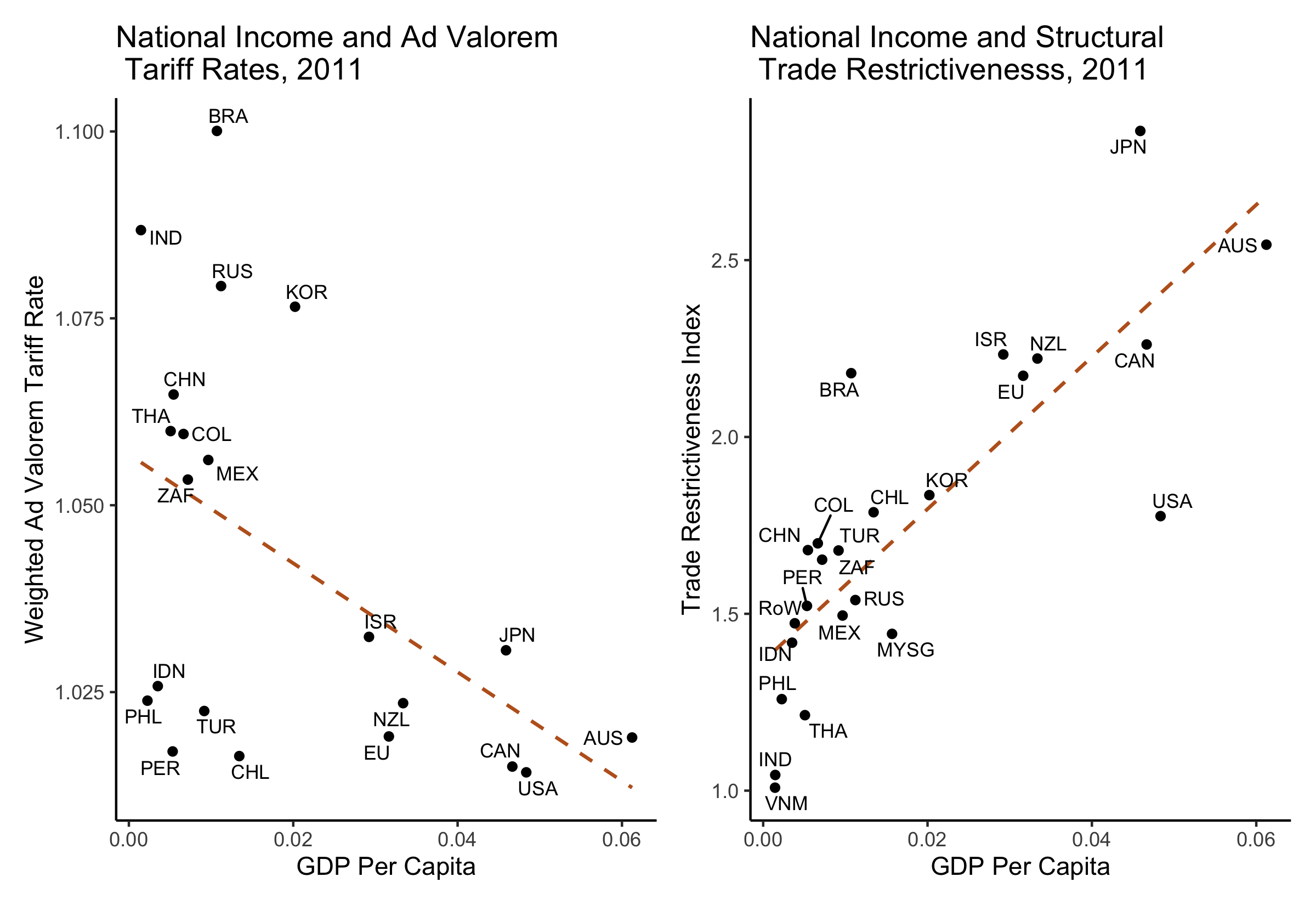## Approach

Trade Costs: Policy distortions and transportation costs to access market $$i$$ in excess of those faced by firms in country $$i$$

Overview

1. Build model of international economy subject to trade costs (Eaton and Kortum 2002)
1. Derive structural relationship linking trade costs to price levels, trade flows, market shares of home producers
• Anderson and Van Wincoop (2003), Waugh (2010), Simonovska and Waugh (2014), Sposi (2015), Waugh and Ravikumar (2016)
1. Decompose trade costs into economic (transportation costs) and political (policy barriers) components
1. Model transportation costs, combine with data on variables in (2) to separately estimate magnitude of policy barriers

$$\tau_{ij} - 1 \geq 0$$ – ad valorem tariff equivalent of policy barriers gov $$i$$ imposes on gov $$j$$

## Identification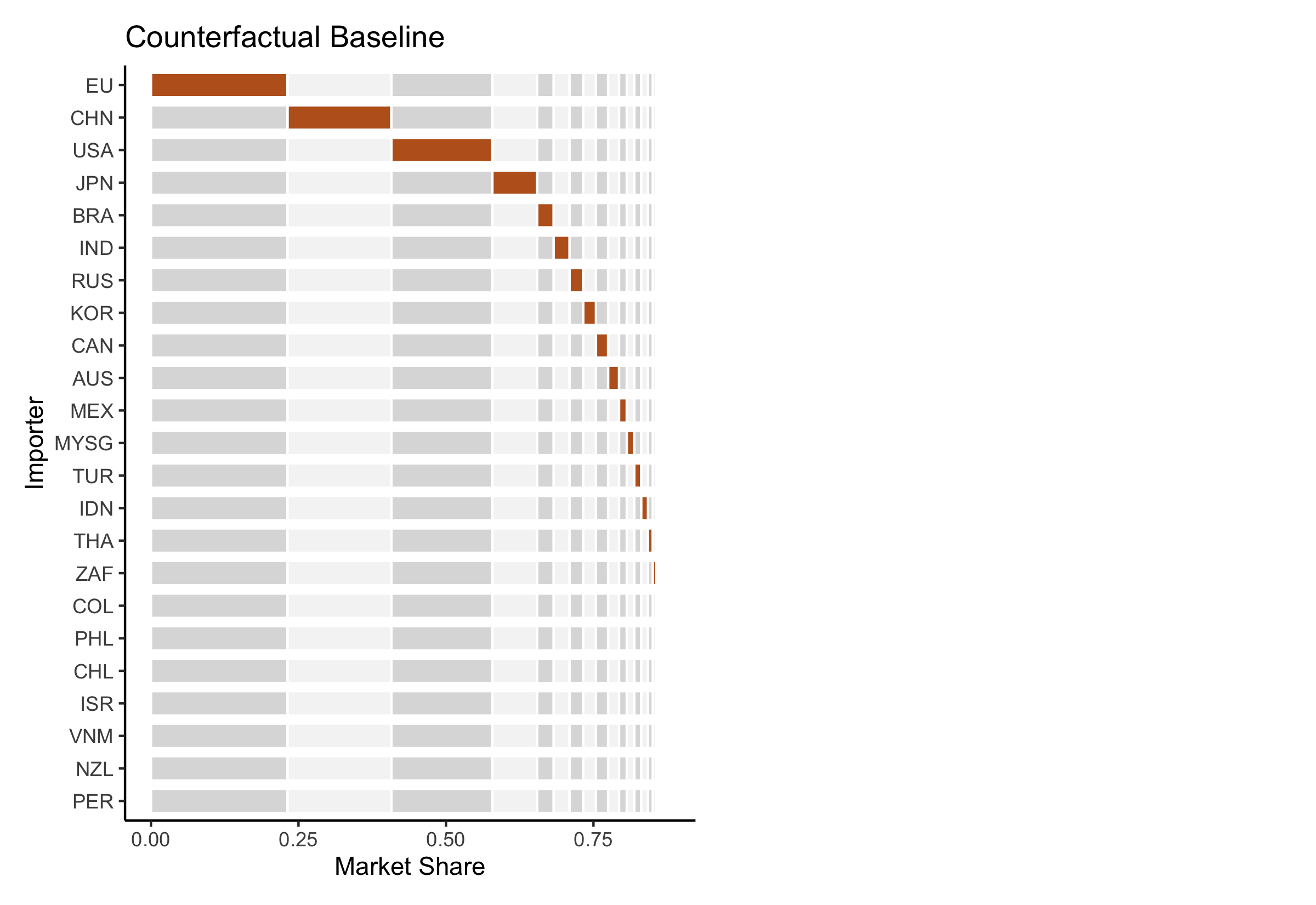## Identification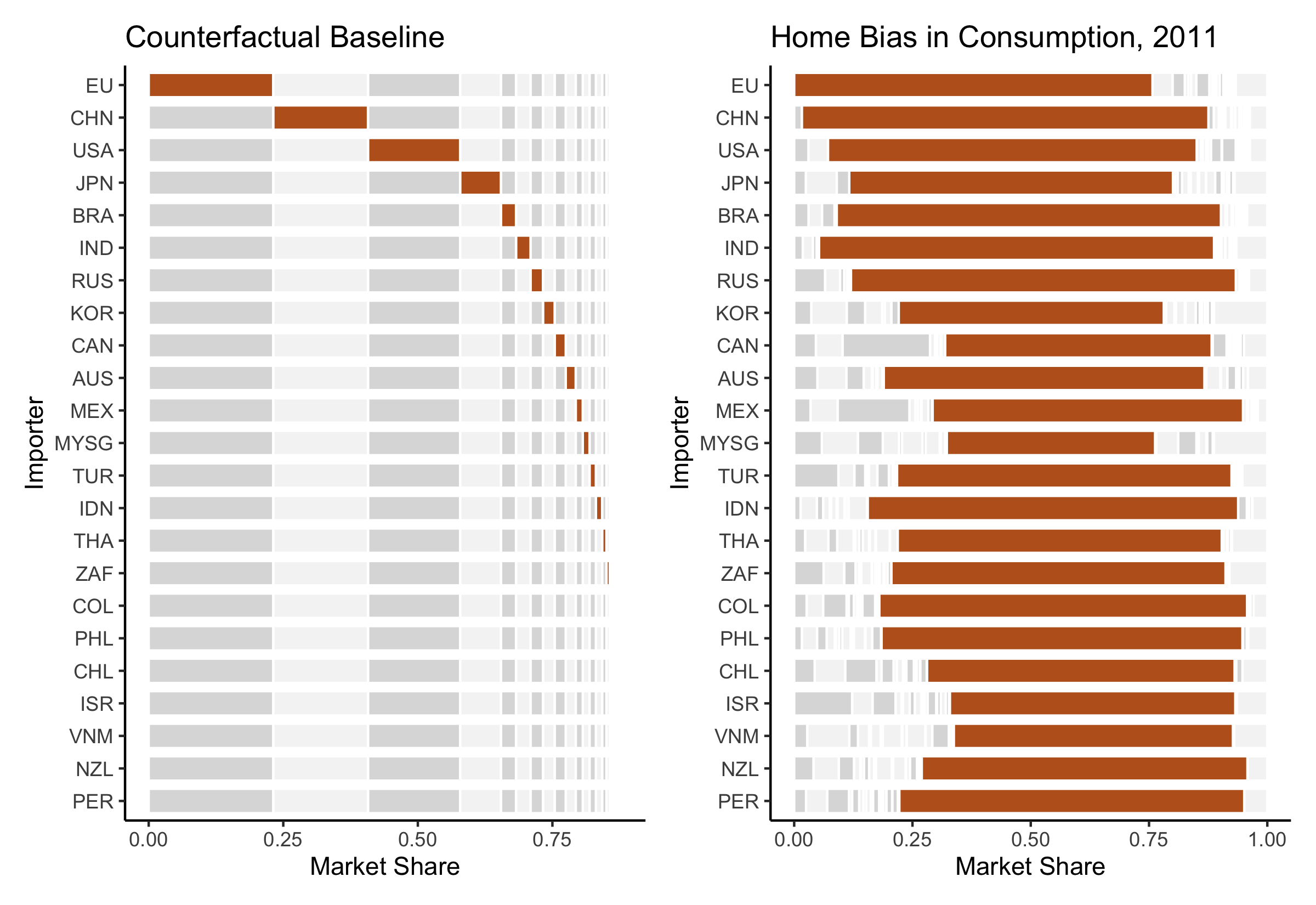## Model Overview

• $$N$$ countries $$i \in \left\{ 1, ..., N \right\}$$ with representative consumers
• Value C-D mixture of tradable goods and nontradable services
• Earn wage $$w_i$$ for every unit of labor endowment $$L_i$$ supplied
• Competitive economy, stochastic technology
• Production requires labor and bundle of intermediate inputs

Structural Relationship

$\begin{equation} \label{eq:Waugh} d_{ij} = \left( \frac{\lambda_{ij}}{\lambda_{jj}} \right)^{-\frac{1}{\theta}} \frac{P_i}{P_j} \end{equation}$

• $$d_{ij}$$ - trade costs
• $$\lambda_{ij}$$ - share of $$j$$’s producers in $$i$$’s market for tradables
• $$\lambda_{jj}$$ - share of $$j$$’s producers in home market for tradables
• $$P_i$$ - price level of tradables
• $$\theta$$ - trade elasticity

Model

## Estimation

Estimating Equation

$\tau_{ij} = \left( \frac{\lambda_{ij}^{\text{cif}}}{\lambda_{jj}} \right)^{-\frac{1}{\theta}} \frac{\hat{P}_i}{\hat{P}_j} \frac{1}{\delta_{ij}(\boldsymbol{Z}_{ij})}$

Math

Data

• Trade shares: national accounts, COMTRADE Shares
• Prices: World Bank International Comparison Program Prices
• Freight Costs: Freight Model
• US Census Bureau, Australian Bureau of Statistics, OECD Maritime Transport Costs
• Estimate out of sample
• Geography (distances, island, contiguity) $$(\boldsymbol{Z}_{ij})$$

## Results (Magnitudes)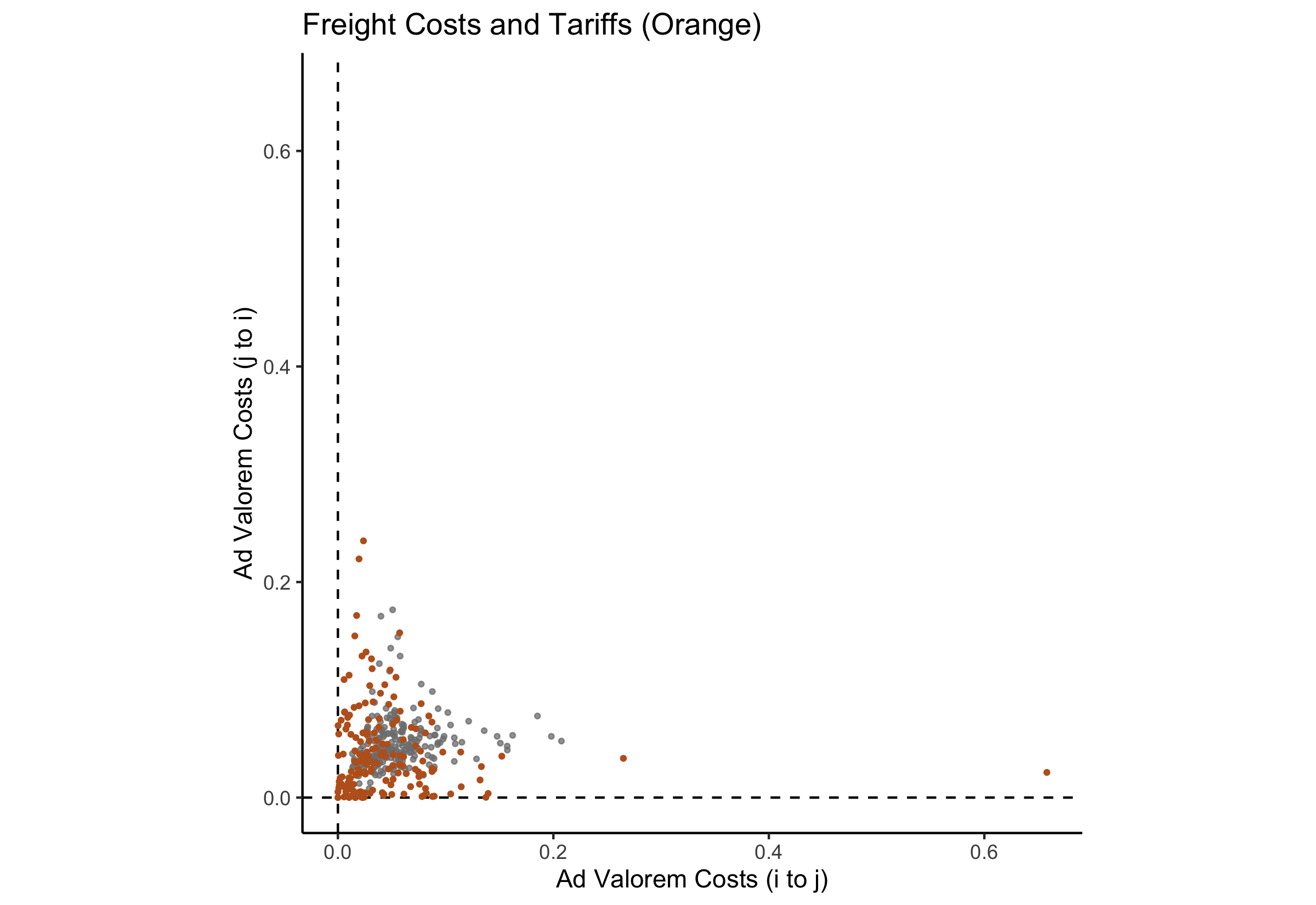## Results (Magnitudes)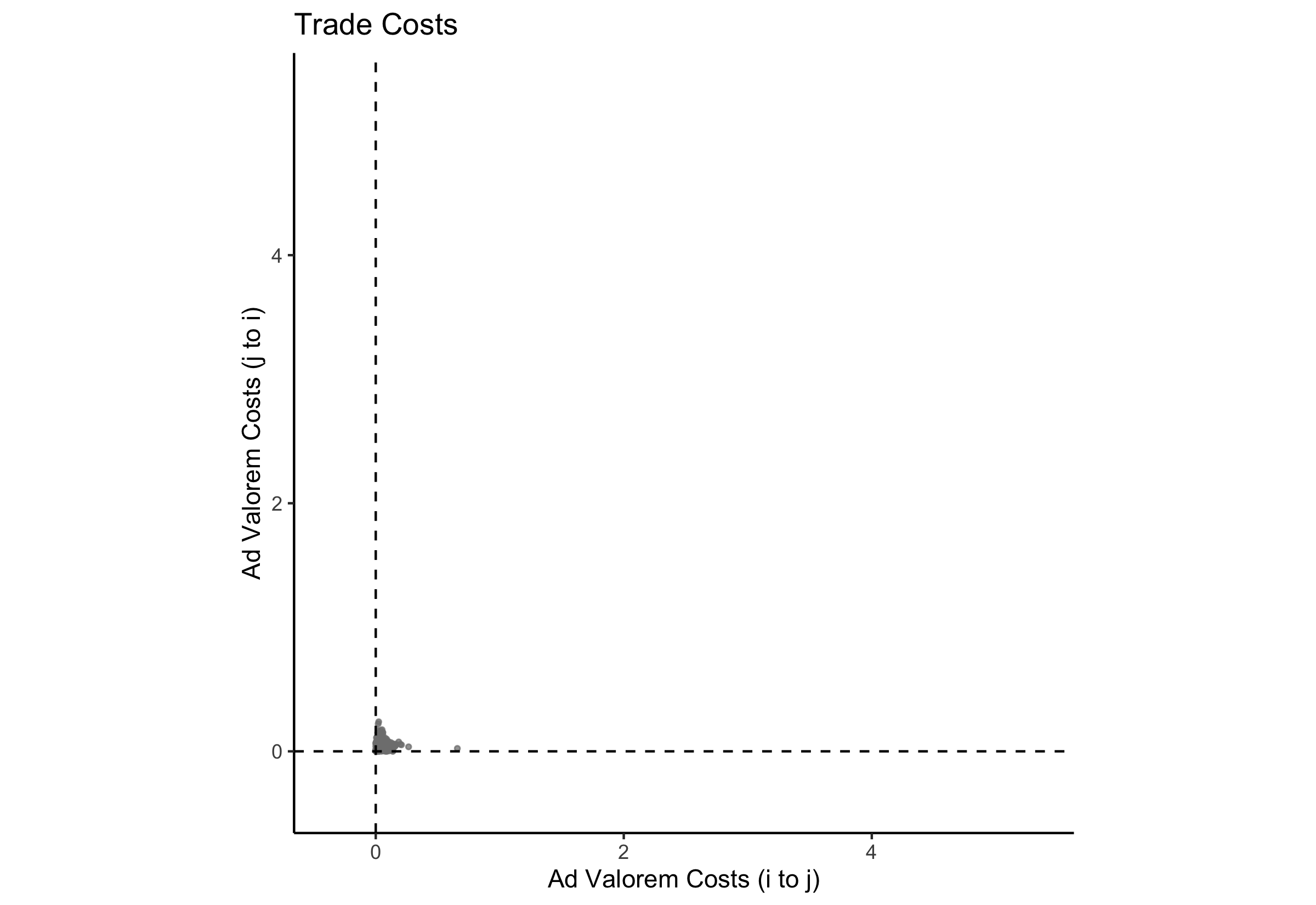## Results (Magnitudes)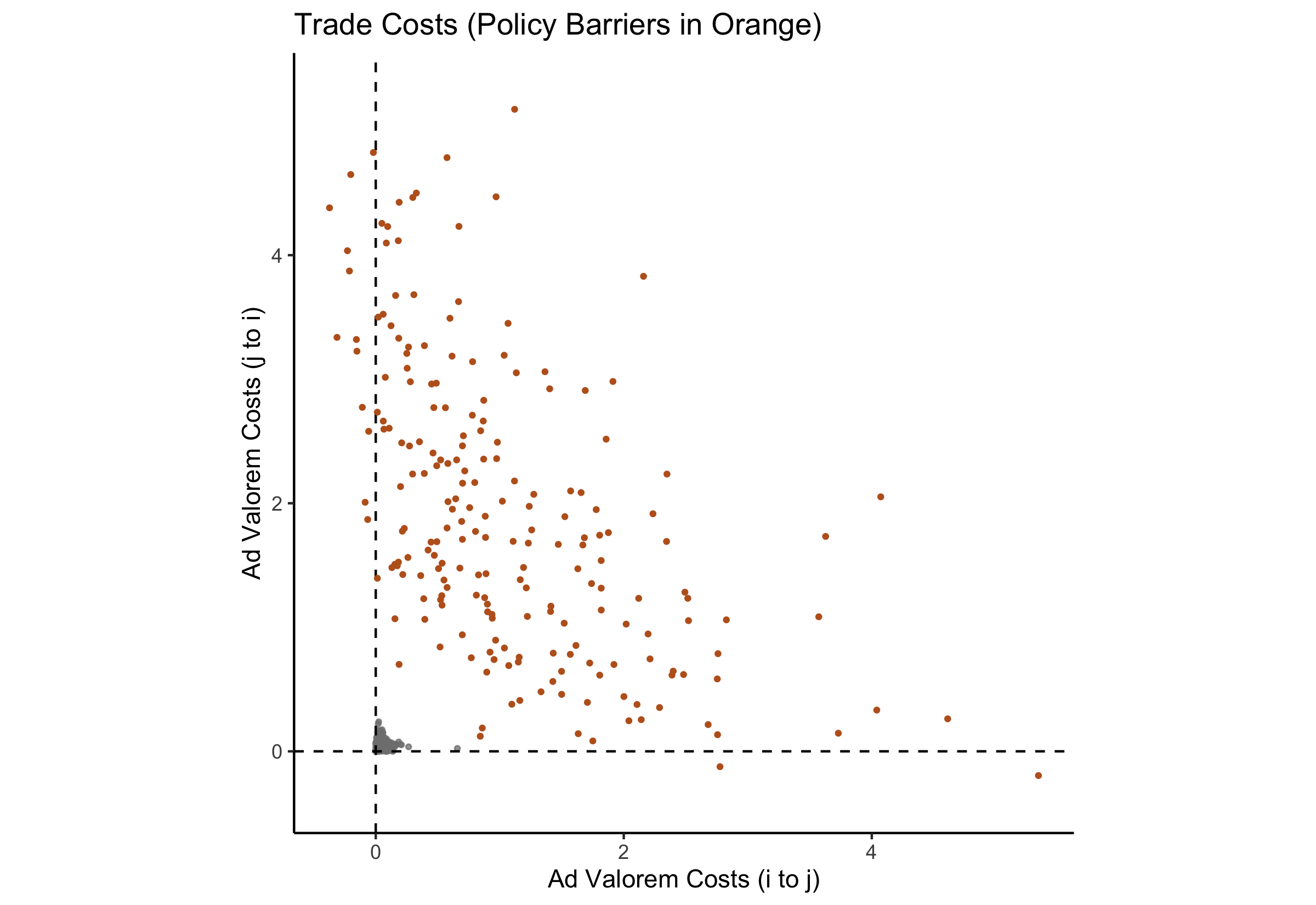## Results (Economic Blocs)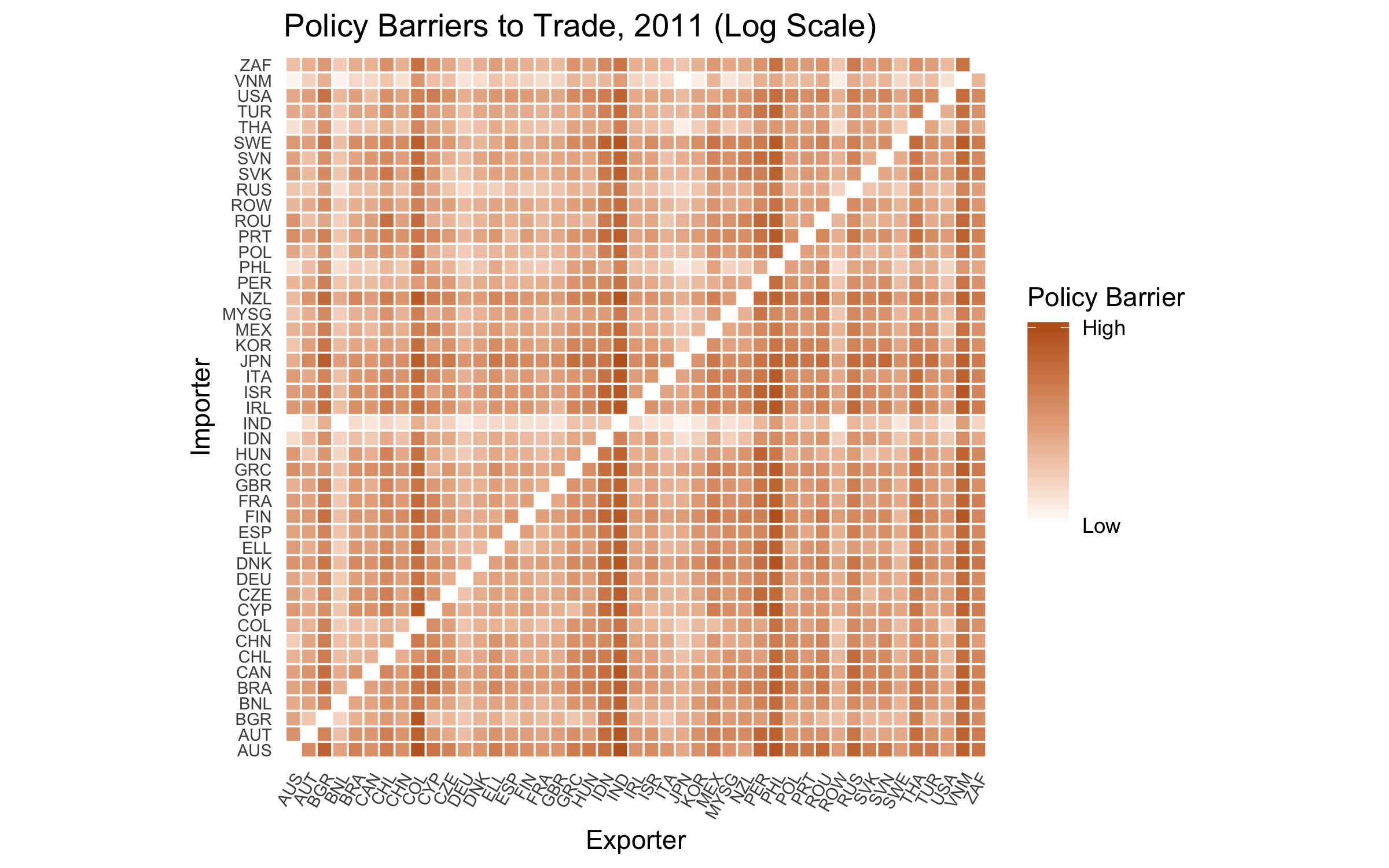EU Placebo

## Correlates of Policy Barriers

 DV: Structural Policy Barrier Tariffs 0.815 (0.738) PTAs -0.376*** (0.082) Core NTM 0.222 (0.211) Health/Safety NTM 0.234 (0.196) Other NTM -0.203 (0.265) Importer Fixed Effects Yes Exporter Fixed Effects Yes N 361 R2 0.892 Notes: ***Significant at the 1 percent level. **Significant at the 5 percent level. *Significant at the 10 percent level.

## Conclusion

State Capacity and Protectionism

• Tariffs as “second-best” solution to revenue-raising problem facing low-capacity governments
• Acemoglu (2005), Rodrik (2008), and Queralt (2015)
• Developing (more autocratic) world not necessarily more “welfare-concious” than developed counterparts
• Milner and Kubota (2005), Gawande, Krishna, and Olarreaga (2009); Gawande, Krishna, and Olarreaga (2015)

Development and Trade Discrimination

• Growth causally linked to market access conditions abroad (Romalis 2007)
• Lack of market access by developing countries may hinder development prospects
• Redding and Venables (2004), Romalis (2007), Waugh (2010)

TRI, MAI

## Thank You

brendancooley.com

[email protected]

## Model (Consumption)

Consumer’s Problem

$\begin{equation*} \label{eq:consumer} \begin{split} \max & \quad U_i = Q_i^{\nu_i} S_i^{1 - \nu_i} \\ \text{subject to} & \quad P_i Q_i + P_i^s S_i \leq w_i L_i \end{split} \end{equation*}$

CES Preferences over Tradable Varieties

$Q_i = \left( \int_{[0,1]} \alpha_{h(\omega)}^{\frac{1}{\sigma}} q_i(\omega)^{\frac{\sigma - 1}{\sigma}} d \omega \right)^{\frac{\sigma}{\sigma - 1}}$

Tradable Price Index

$P_i = \left( \int_{[0,1]} \alpha_{h(\omega)} p_i(\omega)^{1 - \sigma} d \omega \right)^{\frac{1}{1 - \sigma}}$

Expenditure on Tradables

$E_i^q = \nu_i I_i + D_i = P_i Q_i^\star$

Back

## Model (Production)

Costs

$\begin{equation} \label{eq:c} c_i = w_i^{1 - \beta} P_i^{\beta} \end{equation}$

• $$w_i$$ - cost of labor
• $$P_i$$ - cost of composite intermediate good
• equivalent to composite consumption good

$E_i^x = \beta X_i$

Local Prices

$p_{ii}(\omega) = \frac{c_i}{z_i(\omega)}$

Technology (Frechet)

$F_i(z) = \text{Pr} \left\{ z_i(\omega) \leq z \right\} = \exp \left\{ - T_i z^{-\theta} \right\}$

Back

## Model (Equilibrium)

Total Expenditure on Tradables

$X_i = \underbrace{E_i^q + E_i^x}_{E_i} - D_i$

Trade Shares

$\Omega_{ij}^\star = \left\{ \omega \in [0,1] \left. \right\vert p_{ij}(\omega) \leq \min_{k \neq j} \left\{ p_{ik} \right\} \right\}$

$\lambda_{ij}(\boldsymbol{w}) = \frac{1}{E_i} \int_{\Omega_{ij}^\star} p_{ij}(\omega) q_i \left( p_{ij} (\omega) \right) d \omega$

Market Clearing

$X_i = \sum_{j=1}^N \lambda_{ji}(\boldsymbol{w}) E_j$

Back

## Isolating Policy Barriers (I)

Trade Shares (w/ Frechet)

\begin{align*} \lambda_{ij}(\boldsymbol{w}) &= \frac{ T_j \left( d_{ij} w_j^{1 - \beta} P_j^{\beta} \right)^{- \theta} }{\sum_j T_j \left( d_{ij} w_j^{1 - \beta} P_j^{\beta} \right)^{- \theta}} \\ &= \frac{ T_j \left( \underbrace{\rho_j \delta_{ij} \tau_{ij}}_{d_{ij}} w_j^{1 - \beta} P_j^{\beta} \right)^{- \theta} }{ \frac{1}{\gamma} P_i^{-\theta}} \end{align*}

## Isolating Policy Barriers (II)

In Differences

$\frac{\lambda_{ij}}{\lambda_{jj}} = \left( \underbrace{\delta_{ij} \tau_{ij} \frac{P_j}{P_i}}_{\text{observables}}\right)^{- \theta}$

$\tau_{ij} = \left( \frac{\lambda_{ij}}{\lambda_{jj}} \right)^{-\frac{1}{\theta}} \frac{P_i}{P_j} \underbrace{\frac{1}{\delta_{ij}}}_{\substack{\text{freight cost} \\ \text{correction}}}$

Back

## Trade Shares

Behind the Border Barriers

$\lambda_{ij} = \tau_{ij} \lambda_{ij}^{\text{cif}}$

Home Expenditure

$\lambda_{jj}(\boldsymbol{\tau}_j) = \left( 1 - \sum_{i \neq j} \tau_{ji} \lambda_{ji}^{\text{cif}} \right)$

Modified Estimating Equation

$\tau_{ij} = \left( \frac{\lambda_{ij}^{\text{cif}}}{\lambda_{jj}(\boldsymbol{\tau}_j)} \right)^{-\frac{1}{\theta + 1}} \left( \frac{P_i}{P_j} \right)^{\frac{\theta}{\theta+1}} \left( \frac{1}{\delta_{ij}(\boldsymbol{Z}_{ij})} \right)^{\frac{\theta}{\theta+1}}$

Back

## Prices (Model)

• Set$$\mathcal{K}$$ of tradable good categories indexed $$k \in \left\{ 0, ..., K - 1 \right\}$$

$h : \Omega \rightarrow \mathcal{K}$

$\Omega_k = \left\{ \omega : h(\omega) = k \right\}$

Demand

$x_i(\omega) = p_i(\omega) q_i(\omega) = \alpha_{h(\omega)} p_i(\omega)^{1-\sigma} E_i^q P_i^{\sigma - 1}$

Normalization

$\frac{\lambda_{ik}}{\lambda_{i0}} = \alpha_k \left( \frac{p_{ik}}{p_{i0}} \right)^{1 - \sigma}$

Estimation

$\ln \Delta \lambda_{ik} = \ln \alpha_k + (1 - \sigma) \ln \Delta p_{ik} + \ln \epsilon_{ik}$

Back

## Prices (Data)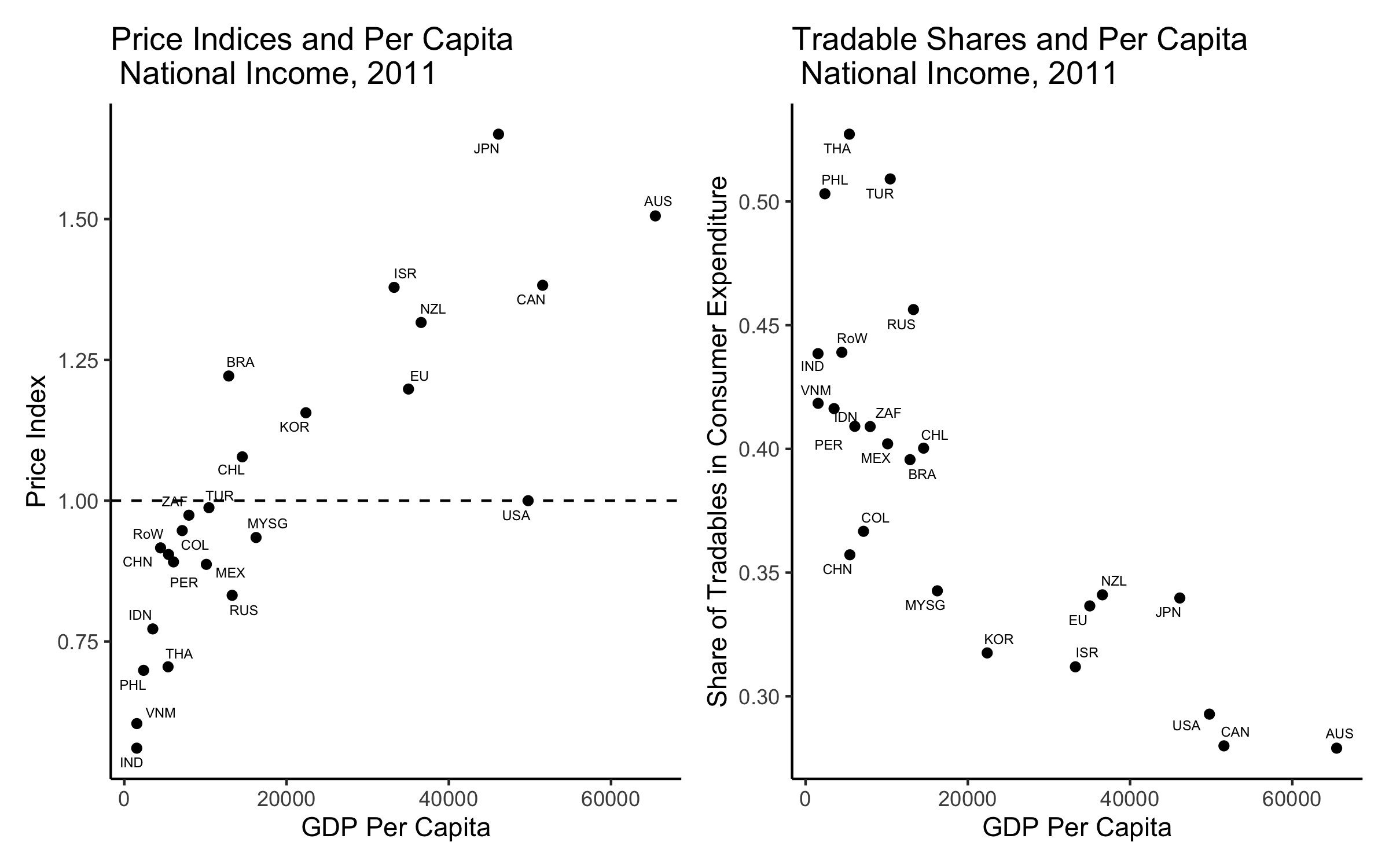Back

## Freight Costs (Model)

• Products $$m \in \left\{ 1, ..., M \right\}$$
• Transportation modes $$k \in \left\{ 1, ..., K \right\}$$
• $$\zeta_{ij}^{m k}$$ share of product $$m$$ transported by mode $$k$$

Mode and Cost Functions

$g: \left\{ \boldsymbol{Z}_{ij}, d^m \right\} \rightarrow \delta_{ij}^{m k}$ $h: \left\{ \boldsymbol{Z}_{ij}, d^m \right\} \rightarrow \zeta_{ij}^{m k}$

Aggregate Freight Costs

$\hat{\delta}_{ij} \left( \boldsymbol{Z}_{ij}, \boldsymbol{d}_{ij} \right) = \frac{1}{X_{ij}} \sum_{m = 1}^M x_{ij}^m \sum_{k=1}^K g \left( \boldsymbol{Z}_{ij}, d^m \right) h \left( \boldsymbol{Z}_{ij}, d^m \right)$

Back

## Freight Costs (Cross Validation)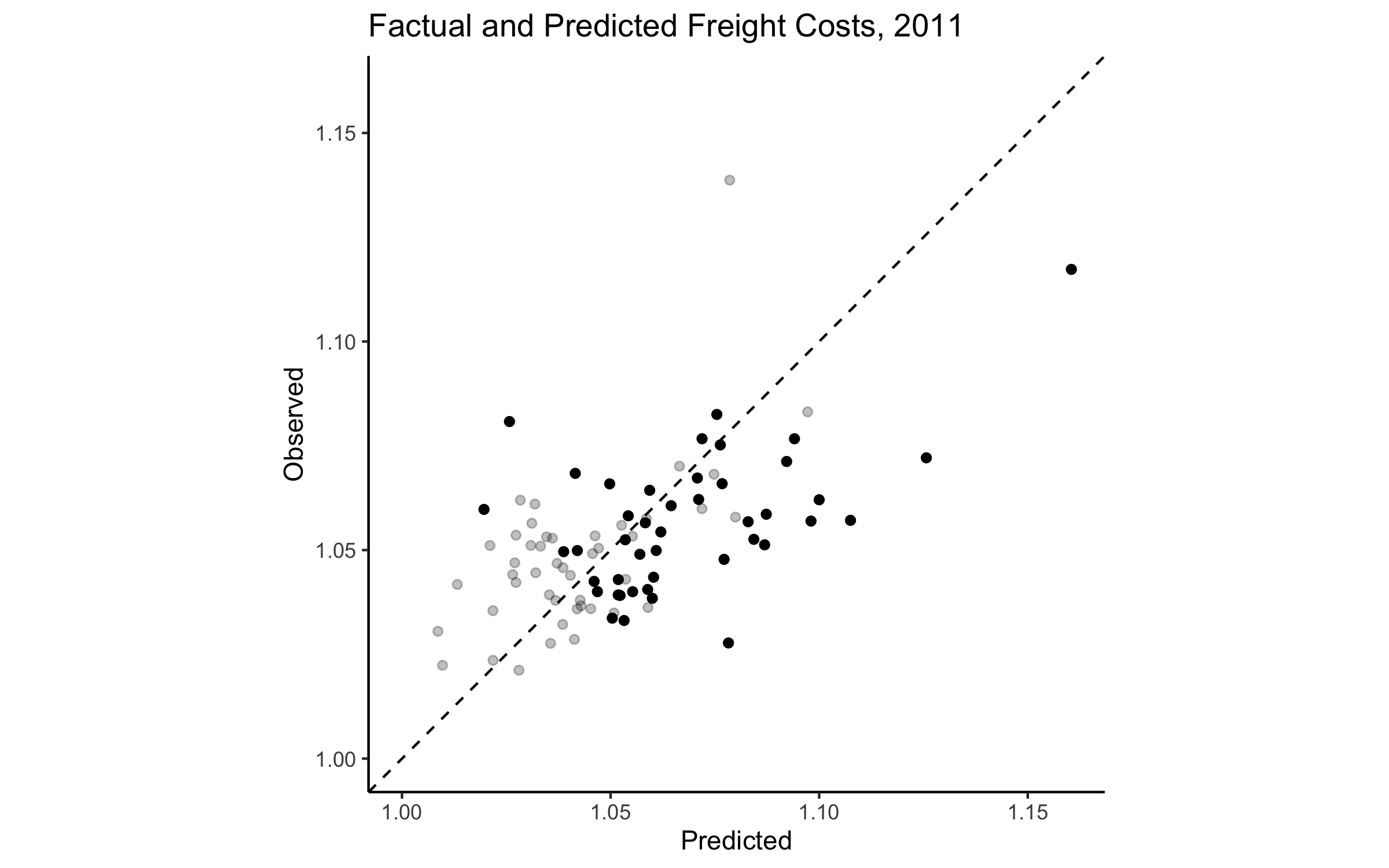Back

## EU Placebo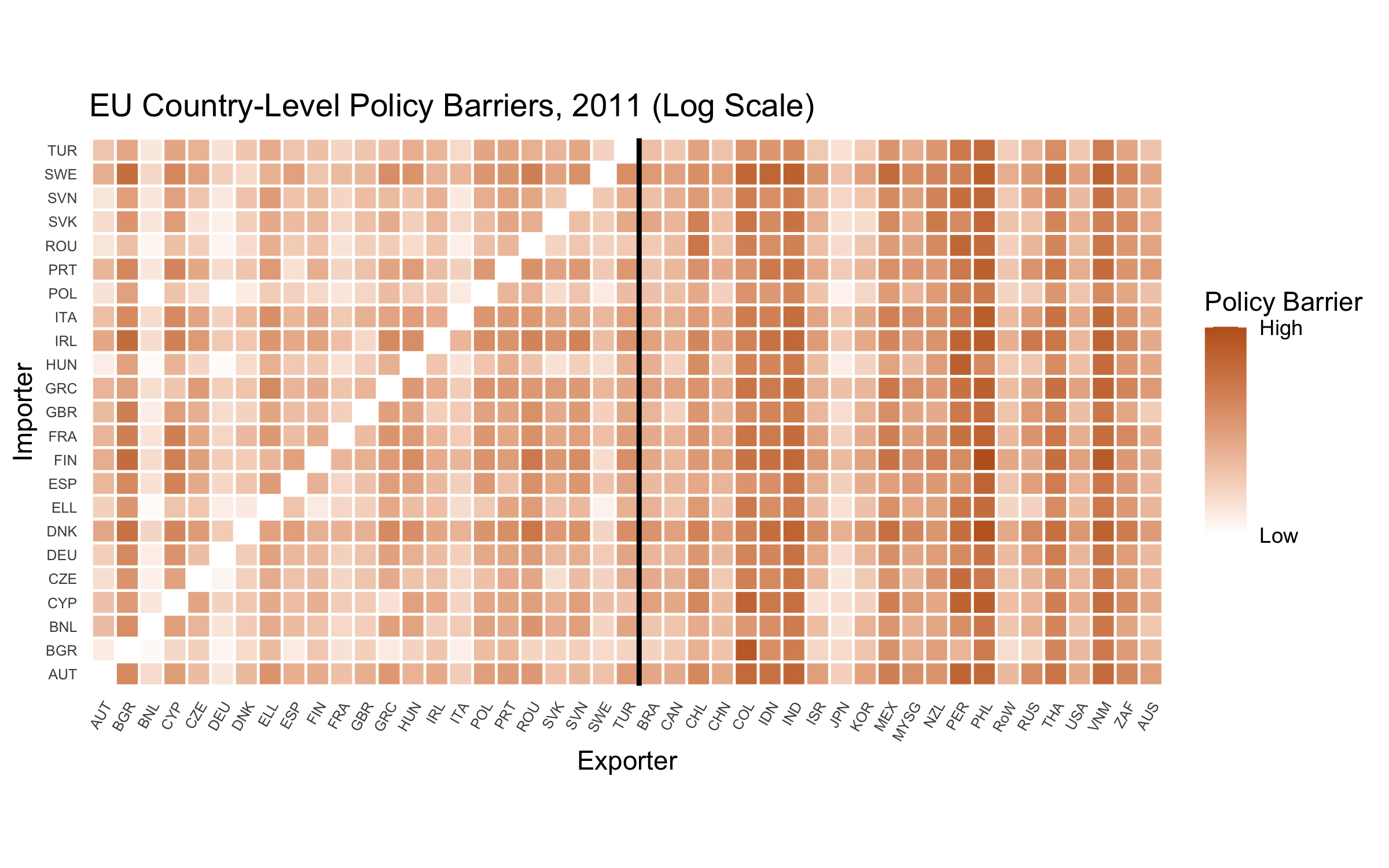Back

## Trade Restrictiveness and Market Access (I)

Trade Restrictiveness Index

$\text{TRI}_i = \frac{1}{\sum_{j \neq i} E_j} \sum_{j \neq i} \tau_{ij} E_j$

Market Access Index

$\text{MAI}_j = \frac{1}{\sum_{i \neq j} E_i} \sum_{i \neq j} \tau_{ij} E_i$

Back

## Trade Restrictiveness and Market Access (II)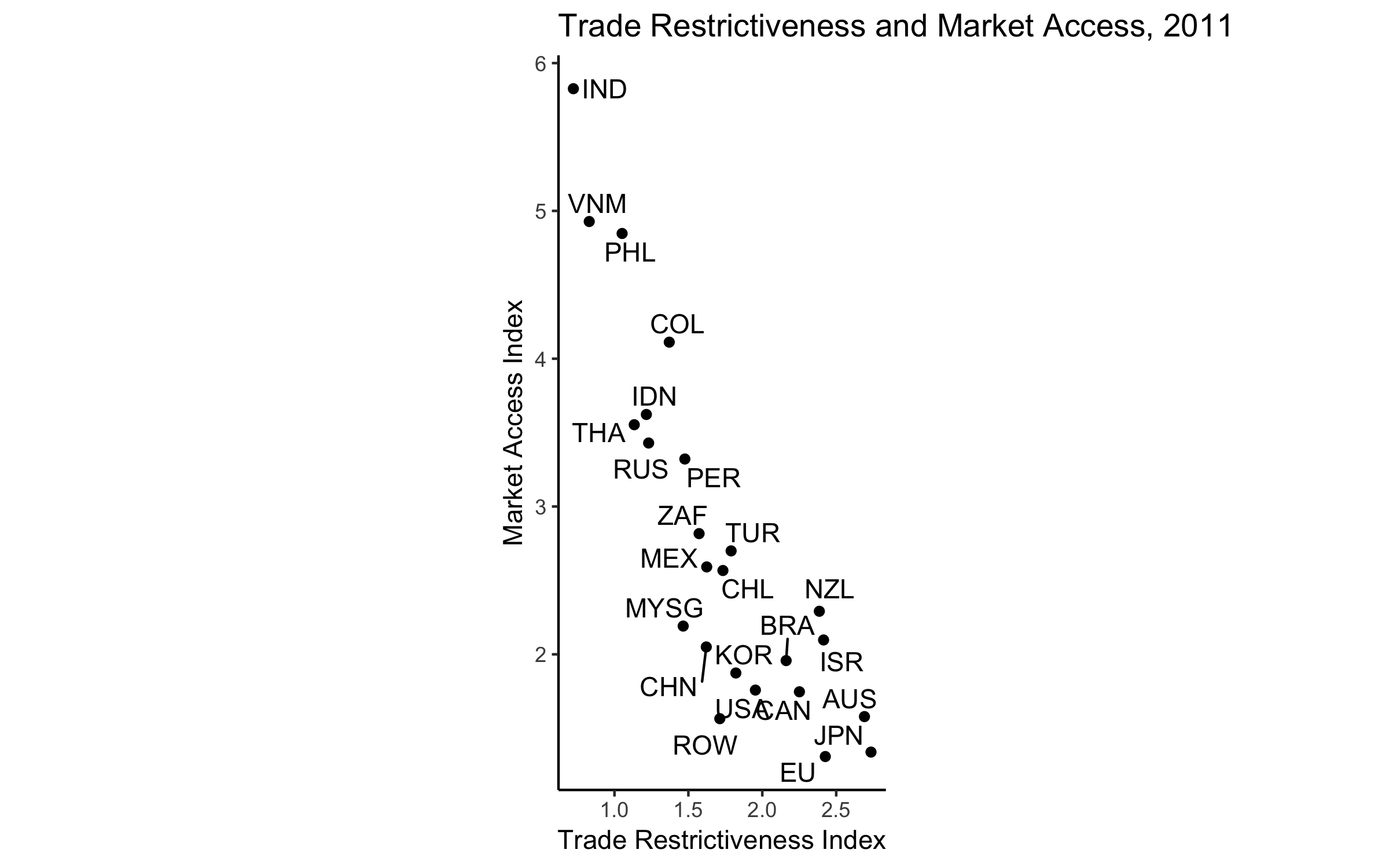Back

## Market Access Barriers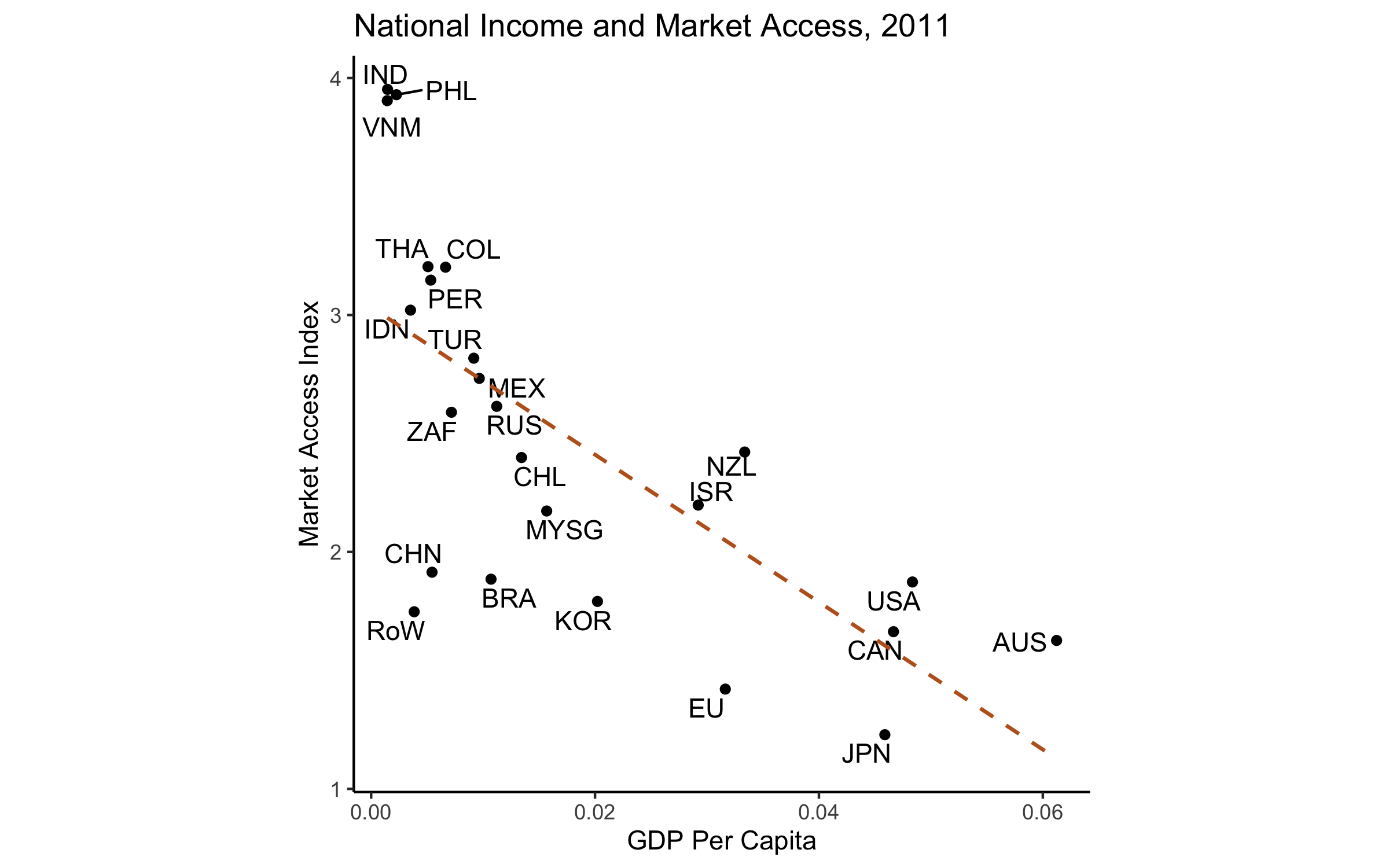Back

Acemoglu, Daron. 2005. “Politics and economics in weak and strong states.” Journal of Monetary Economics 52 (7): 1199–1226.

Anderson, James E, and Eric Van Wincoop. 2003. “Gravity with Gravitas: A Solution to the Border Puzzle.” The American Economic Review 93 (1): 170–92.

Baldwin, Richard. 2016. “The World Trade Organization and the Future of Multilateralism.” Journal of Economic Perspectives 30: 95–116.

Eaton, Jonathan, and Samuel Kortum. 2002. “Technology, geography, and trade.” Econometrica 70 (5): 1741–79.

Evenett, Simon J., and Bernard Hoekman. 2004. Government Procurement: Market Access, Transparency, and Multilateral Trade Rules. Policy Research Working Papers. The World Bank.

Gawande, Kishore, and Wendy L. Hansen. 1999. “Retaliation, Bargaining, and the Pursuit of "Free and Fair" Trade.” International Organization 53 (1): 117–59.

Gawande, Kishore, Pravin Krishna, and Marcelo Olarreaga. 2009. “What governments maximize and why: the view from trade.” International Organization 63 (03): 491–532.

———. 2015. “A Political-Economic Account of Global Tariffs.” Economics & Politics 27 (2): 204–33.

Kono, Daniel Yuichi. 2006. “Optimal obfuscation: Democracy and trade policy transparency.” American Political Science Review 100 (03): 369–84.

Kono, Daniel Yuichi, and Stephanie J Rickard. 2014. “Buying National: Democracy, Public Procurement, and International Trade.” International Interactions 40 (5): 657–82.

Lee, Jong-Wha, and Phillip Swagel. 1997. “Trade Barriers and Trade Flows Across Countries and Industries.” The Review of Economics and Statistics 79 (3): 372–82.

Maggi, Giovanni, Monika Mrázová, and J Peter Neary. 2018. “Choked by Red Tape? The Political Economy of Wasteful Trade Barriers.”

Mansfield, Edward D., and Marc L. Busch. 1995. “The Political Economy of Nontariff Barriers: A Cross-National Analysis.” International Organization 49 (4): 723–49.

Milner, Helen V, and Keiko Kubota. 2005. “Why the move to free trade? Democracy and trade policy in the developing countries.” International Organization 59 (01): 107–43.

Queralt, Didac. 2015. “From Mercantilism to Free Trade: A History of Fiscal Capacity Building.” Quarterly Journal of Political Science 10: 221–73.

Redding, Stephen, and Anthony J Venables. 2004. “Economic geography and international inequality.” Journal of International Economics 62: 53–82.

Rickard, Stephanie J. 2012. “A Non-Tariff Protectionist Bias in Majoritarian Politics: Government Subsidies and Electoral Institutions.” International Studies Quarterly 56: 777–85.

Rodrik, Dani. 2008. “Second-Best Institutions.” American Economic Review: Papers & Proceedings 98 (2): 100–104.

Romalis, John. 2007. “Market Access, Openness and Growth.” Cambridge, MA: National Bureau of Economic Research.

Simonovska, Ina, and Michael E Waugh. 2014. “The Elasticity of Trade: Estimates and Evidence.” Journal of International Economics 92 (1): 34–50.

Sposi, Michael. 2015. “Trade barriers and the relative price of tradables.” Journal of International Economics 96 (2): 398–411.

Waugh, Michael E. 2010. “International Trade and Income Differences.” American Economic Review 100: 2093–2124.

Waugh, Michael E, and B Ravikumar. 2016. “Measuring Openness to Trade.” Journal of Economic Dynamics and Control 72: 29–41.